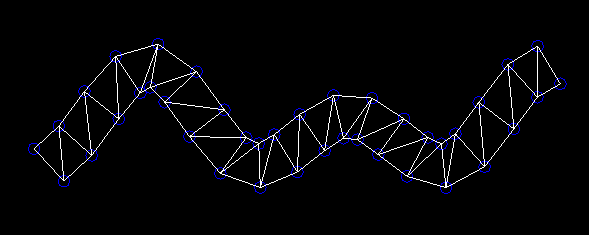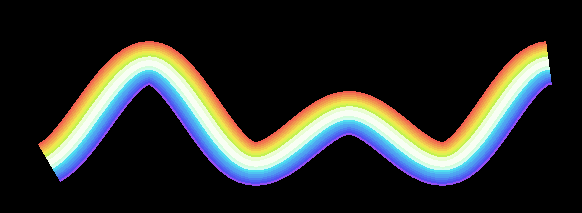# libGDX How can I draw texture parallel to edge in polygon?

I’m trying to make polyline with thickness.
From point I need to connect I generate polygon(image 1~5)
I want texture to be filled in polygon.
So I used EarClippingTriangulator to cut polygon and declare ‘PolygonRegion’.(image 6)
But when I draw PolygonRegion result is not what I want.(image 7-a)
I want polygon to be filled with texture parallel to edge (image 7-b)
How can I do this in libgdx?

you need to give specific texture coordinates to each vertex, based on their distance from the center of the line rather than their x,y position. although that triangulated polygon might give deformed texturing, so you also might need to triangulate it yourself with more evenly placed triangles - fairly easy by extruding triangles on either side of the center line. (ShapeRenderer is a useful class for testing/drawing triangles if it is awkward to work with PolygonRegion in this way)

Ok, I’ve changed how it is triangulated.But How can I give specific coordinate to each vertex?
I assume method
PolygonSpriteBatch.draw(texture, polygonVertices, verticesOffset, verticesCount, polygonTriangles, trianglesOffset, trianglesCount);
is how it’s done but, googling this method doesn’t get any result.
API says ‘There must be 4 vertices, each made up of 5 elements in this order: x, y, color, u, v.’
But I don’t understand what are those vertices and how it should be generated.
Any simple guideline on how to approach this would be appreciated.

have a look at the source code for some of the SpriteBatch.draw() methods, they basically work the same way - by putting the vertex data into a float[] array which is eventually sent to the gpu for rendering, except they calculate the vertex data automatically for you instead of requiring you to specify the data for each vertex yourself (which is what you do want to do in this case).

It took longer than expected but I finally figured out how to do it.
Thanks to your answer, my game can now have beautiful Rainbow Beam attack!For anyone may have similar problem with me, I’ll leave simple code here.

‘vertices’ is float array of vertices from given polygon I’m try to fill in.
vertices is generated top-left corner to bottom-left corner in clockwise order.

``````

private float[] testVertices;
private short[] testTriangles;
private Texture testImage;
public void manualTriangulor() {
testVertices=new float[(vertices.length-4)*5];
testTriangles=new short[(vertices.length/2-2)*3];
for(int i=0; i<vertices.length/4-1; i++) {
testVertices[i*20]=vertices[((vertices.length/2)-i-1)*2];
testVertices[i*20+1]=vertices[((vertices.length/2)-i-1)*2+1];
testVertices[i*20+2]=Color.WHITE.toFloatBits();
testVertices[i*20+3]=0;
testVertices[i*20+4]=1;

testVertices[i*20+5]=vertices[i*2];
testVertices[i*20+6]=vertices[i*2+1];
testVertices[i*20+7]=Color.WHITE.toFloatBits();
testVertices[i*20+8]=0;
testVertices[i*20+9]=0;

testVertices[i*20+10]=vertices[(i+1)*2];
testVertices[i*20+11]=vertices[(i+1)*2+1];
testVertices[i*20+12]=Color.WHITE.toFloatBits();
testVertices[i*20+13]=1;
testVertices[i*20+14]=0;

testVertices[i*20+15]=vertices[((vertices.length/2)-i-2)*2];
testVertices[i*20+16]=vertices[((vertices.length/2)-i-2)*2+1];
testVertices[i*20+17]=Color.WHITE.toFloatBits();
testVertices[i*20+18]=1;
testVertices[i*20+19]=1;

testTriangles[i*6]=(short)(i*4);
testTriangles[i*6+1]=(short)(i*4+1);
testTriangles[i*6+2]=(short)(i*4+2);

testTriangles[i*6+3]=(short)(i*4+2);
testTriangles[i*6+4]=(short)(i*4+3);
testTriangles[i*6+5]=(short)(i*4);
}
}

public void draw(PolygonSpriteBatch batch) {
batch.begin();
batch.draw(testImage,testVertices,0,testVertices.length,testTriangles,0, testTriangles.length);
batch.end();
}

``````